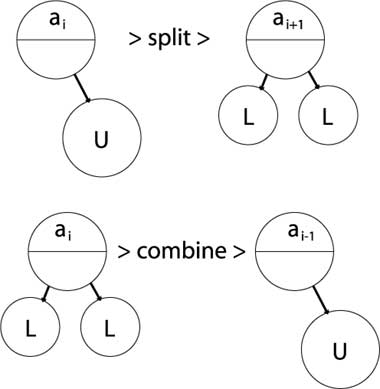# 2.3. B-trees

In this lecture we look at...
[Section notes PDF 159Kb]

# 2.3.01. Hash tables

• Used to implement Indicies
• O(n) access
• Ordering Key Field (K) as argument to Hash function H()
• Address H(K) maps to pointer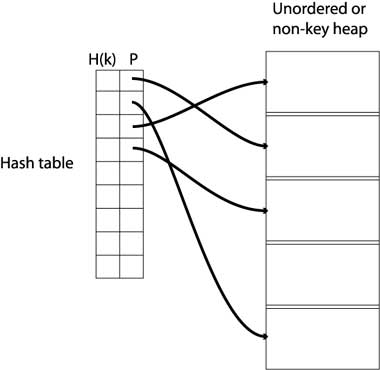# 2.3.02. Tree structure

• Tree revision
• Node based
• Branching nodes/leaf nodes
• Parent/child nodes
• Root node
• Cardinality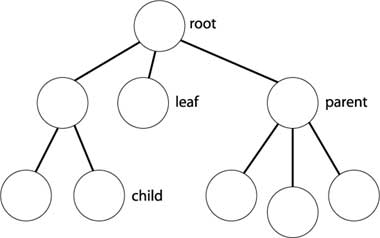# 2.3.03. Multi-level indices

• Multi-level indices
• One index indexes another
• Implemented by multiple hash-tables
• <H(k),P> pairs
• (data far right)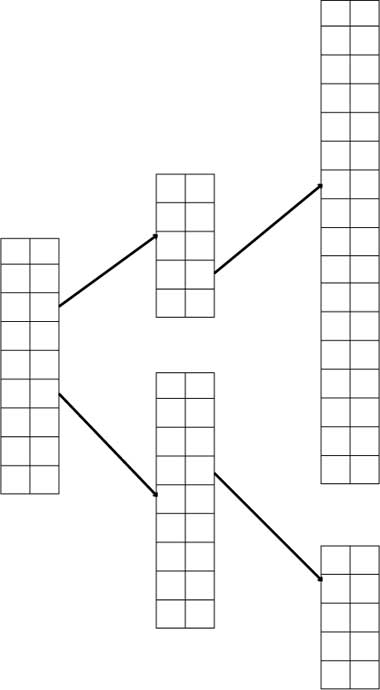# 2.3.04. Index zipping

• Collapsing a single index
• Two columns become one
• <H(k),P> pairs sequentially stored
• Common in the Elmasri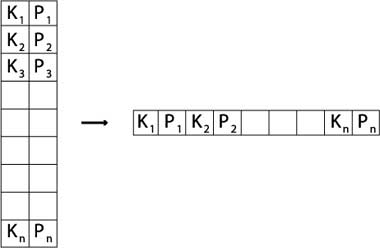# 2.3.05. B-tree

• Paritioning structure
• Each node contains keys & pointers
• Pointers can be:
• Node pointers - to child nodes
• Data pointers - to records in heap
• Number of keys = Number of pointers - 1
• Every node in the tree is identical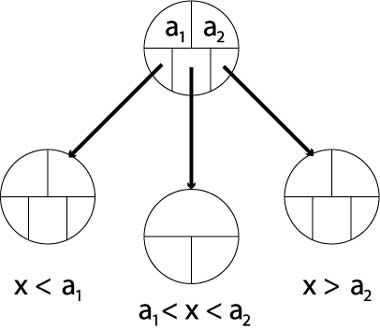# 2.3.06. B+ trees

• Similar to B-trees
• Different types of nodes
• Branching nodes
• Leaf nodes
• Each branching node has:
• At most U children (max U)
• At least L children (min L)
• U = 2L, or U = 2L-1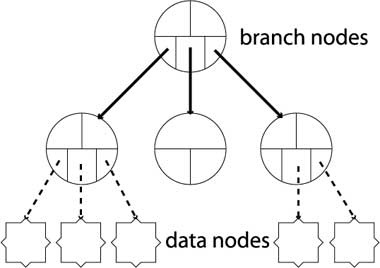# 2.3.07. Properties of B+ trees

• Balanced
• All leaf nodes at same level
• Record search takes same time for every record
• Partitioning needs to be comprehensive
• B-tree: a1 < x < a2
• B+tree: a1 <= x <= a2
• Why?
• because all data for partition values must be in the lowest level of the tree

# 2.3.08. B+ tree operations

• Insert operation cascades from bottom
• Rules: node can contain U children (max)
• Node combine
• Legal if child nodes contain L children
• Parent loses one key/paritition value
• Node split
• Legal if node contains U children
• Parent node gains one key/partition value
• Can cause cascade up tree & rebalancing